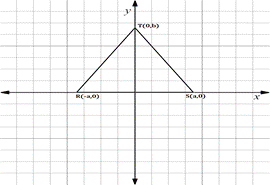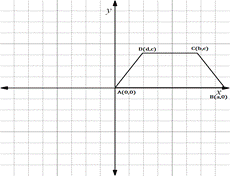Chapter 10.CT, Problem 11CT### Elementary Geometry for College St...

6th Edition
Daniel C. Alexander + 1 other
ISBN: 9781285195698

#### Solutions

Chapter
Section### Elementary Geometry for College St...

6th Edition
Daniel C. Alexander + 1 other
ISBN: 9781285195698
Textbook Problem
6 views

# Being as specific as possible, describe the type of polygon shown in each figure.(a) _____(b) ______

To determine

(a)

To describe:

The type of polygon shown in the given figure.

Explanation

The given figure is shown below.

Find the distance RT.

x1,y1=R-a, 0

x2,y2=T0, b

Formula for distance between two points

d=x2-x12+y2-y12

Substituting the x and y co-ordinates

dRT=0--a2+b-02

On solving this,

dRT=a2+b2

To determine

(b)

To describe:

The type of polygon shown in the given figure.

### Still sussing out bartleby?

Check out a sample textbook solution.

See a sample solution

#### The Solution to Your Study Problems

Bartleby provides explanations to thousands of textbook problems written by our experts, many with advanced degrees!

Get Started

#### Convert the expressions in Exercises 6584 to power form. 25x3

Finite Mathematics and Applied Calculus (MindTap Course List)

#### Convert the following percents to decimals. 422%

Contemporary Mathematics for Business & Consumers

#### Evaluate the integrals in Problems 1-26.

Mathematical Applications for the Management, Life, and Social Sciences

#### For y = x3 ln x, y = a) x2(1 + 3 ln x) b) x3 + 3x2 (ln x) c) 3x d) 4x2(ln x)

Study Guide for Stewart's Single Variable Calculus: Early Transcendentals, 8th

#### What are the five steps of the scientific method?

Research Methods for the Behavioral Sciences (MindTap Course List)